# NMath User's Guide

44.3 Two-Way Balanced ANOVA (.NET, C#, CSharp, VB, Visual Basic, F#)

Class performs a balanced two-way analysis of variance. Two-way analysis of variance is a direct extension of one-way analysis of variance (Section 44.1). In this case, data are grouped according to two factors—for example, sex and age group—rather than a single factor. The total variability is partitioned into components associated with each of the two factors, their interaction, and the residual (or error).

Creating Two-Way ANOVA Objects

A TwoWayAnova instance is constructed from data in a data frame. Three column indices are specified in the data frame: the column containing the first factor, the column containing the second factor, and the column containing the numeric data. For example, this code groups the numeric data in column 3 of DataFrame df by factors constructed from columns 0 and 4:

Code Example – C# ANOVA

```var anova = new TwoWayAnova( df, 0, 4, 3 );
```

Code Example – VB ANOVA

```Dim Anova As New TwoWayAnova(DF, 0, 4, 3)
```

Factor objects are constructed from the factor columns using the DataFrame method GetFactor(), which creates a sorted array of the unique values (Section 37.10). The indicated data column must be of type DFNumericColumn.

NOTE—Class TwoWayAnova throws an InvalidArgumentException if the data contains missing values (NaNs).

The Two-Way ANOVA Table

Once you've constructed a TwoWayAnova, you can display the complete ANOVA table:

Code Example – C# ANOVA

```Console.WriteLine( anova );
```

Code Example – VB ANOVA

```Console.WriteLine(Anova)
```

For example:

```Source  Deg of Freedom  SumOfSq    Mean Square  F         P
```
```FactorA      1          1782.0450  1782.0450    14.2121   0.0008
```
```FactorB      1          2838.8113  2838.8113    22.6399   0.0001
```
```Interaction  1          108.0450   108.0450     0.8617    0.3612
```
```Error        28         3510.9075  125.3896     .         .
```
```Total        31         8239.8088  .            .         .
```

Class is provided for summarizing the information in a traditional two-way ANOVA table. Class TwoWayAnovaTable derives from DataFrame. An instance of TwoWayAnovaTable can be obtained from a TwoWayAnova object using the AnovaTable property. For example:

Code Example – C# ANOVA

```TwoWayAnovaTable myTable = anova.AnovaTable;
```

Code Example – VB ANOVA

```Dim MyTable As TwoWayAnovaTable = Anova.AnovaTable
```

Class TwoWayAnovaTable provides the following member functions and read­-only properties for accessing individual elements in the ANOVA table:

DegreesOfFreedom() gets the degrees of freedom for a specified factor.

ErrorDegreesOfFreedom gets the number of degrees of freedom for the error.

InteractionDegreesOfFreedom gets the number of degrees of freedom for the interactions.

TotalDegreesOfFreedom gets the total number of degrees of freedom.

SumOfSquares() gets the sum of squares for a specified factor.

InteractionSumOfSquares gets the sum of squares for the interaction.

ErrorSumOfSquares gets the sum of squares for the error.

TotalSumOfSquares gets the total sum of squares.

MeanSquare() gets the mean square for a specified factor.

InteractionMeanSquare gets the mean square for the interaction.

ErrorMeanSquare gets the mean square for the error.

Fstatistic() gets the F statistic for a specified factor.

InteractionFstatistic gets the F statistic for the interaction.

FstatisticPvalue() gets the p-value for the F statistic for a specified factor.

InteractionFstatisticPvalue gets the p-value for the F statistic for the interaction.

Factors are identified to accessor methods by name, which corresponds to the name of the column in the original data frame that was used to create the Factor. For instance, if one factor in the ANOVA is named Dosage, this code gets the statistic and p­-value for that factor:

Code Example – C# ANOVA

```double Fstatistic = anova.AnovaTable.Fstatistic( "Dosage" );
```
```double Pvalue = anova.AnovaTable.FstatisticPvalue( "Dosage" );
```

Code Example – VB ANOVA

```Dim FStatistic As Double = Anova.AnovaTable.FStatistic("Dosage")
```
```Dim PValue As Double = Anova.AnovaTable.FStatisticPValue("Dosage")
```

Cell Data

Class TwoWayAnova provides the GetCellData() method for accessing the data in a cell, as defined by a specified level of each of the factors in the ANOVA. For example, if anova has factor Sex with levels Male and Female, and factor AgeGroup with levels Child, Adult, and Senior, this code gets the data for adult females:

Code Example – C# ANOVA

```DFNumericColumn data =
```
```  anova.GetCellData( "Sex", "Female", "AgeGroup", "Adult" );
```

Code Example – VB ANOVA

```Dim Data As DFNumericColumn =
```
```  Anova.GetCellData("Sex", "Female", "AgeGroup", "Adult")
```

A copy of the data is returned as a DFNumericColumn object.

Grand Mean, Cell Means, and Group Means

Class TwoWayAnova provides the following properties and member functions for accessing the grand mean, cell means, and group means:

GrandMean gets the grand mean. The grand mean is the mean of all the data.

GetMeanForCell() returns the mean for a specified cell.

GetMeanForFactorLevel() returns the mean for a specified factor level.

Again, factors and factor levels are identified to accessor methods by name. For example, if anova has factor Sex with levels Male and Female, and factor AgeGroup with levels Child, Adult, and Senior, this code gets the mean for all males:

Code Example – C# ANOVA

```double meanM = anova.GetMeanForFactorLevel( "Sex", "Male" );
```

Code Example – VB ANOVA

```Dim MeanM As Double = Anova.GetMeanForFactorLevel("Sex", "Male")
```

This code gets the mean for male children:

Code Example – C# ANOVA

```double meanMChild =
```
```  anova.GetMeanForCell( "Sex", "Male", "AgeGroup", "Child" );
```

Code Example – VB ANOVA

```Dim MeanMChild As Double =
```
```  Anova.GetMeanForCell("Sex", "Male", "AgeGroup", "Child")
```

ANOVA Regression Parameters

NMath Stats solves the two-way ANOVA problem using multiple linear regression. If all you wish to know is the information in the standard ANOVA table, you can safely ignore the regression details, but properties and member functions are provided for retrieving information about the underlying regression parameters.

To solve the two-way ANOVA problem using multiple linear regression, NMath Stats creates a series of dummy variables to encode the different levels of each of the two factors. The specific encoding used, known as effects encoding, encodes dummy variables so that the coefficients of the dummy variables in the regression model quantify deviations of each group from the grand mean.1

In the effects encoding,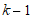dummy variables are defined to encode the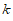levels of a factor, like so: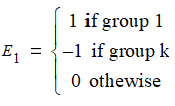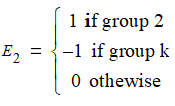and so on, up to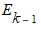for group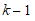.

For example, suppose we have an experimental design with two factors: FactorA and FactorB. FactorA has two levels, labelled A1 and A1. Effects encoding defines one dummy variable for FactorA: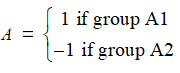FactorB has three levels, labelled B1, B2, and B3. Effects encoding defines two dummy variable for FactorB:Combined, these three dummy variables completely identify all the combinations of FactorA and FactorB. The multiple regression model is then:where

the intercept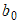is an estimate of the grand meanestimates the difference between the grand mean and the mean of A1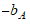is the difference between the grand mean and the mean of A2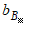estimates the difference between the grand mean and the mean of B1estimates the difference between the grand mean and the mean of B2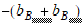estimates the difference between the grand mean and the mean of B3

NMath Stats includes several classes that derive from LinearRegressionParameter, and provide access to the dummy variable regression parameters in an ANOVA analysis of variance:

Class provides a SumOfSquares property that gets the sum of squares due to a parameter.

Class derives from AnovaRegressionParameter and provides the additional properties FactorName, which gets the name of the ANOVA factor encoded by a dummy variable, FactorLevel, which gets the level of the ANOVA factor encoded by a dummy variable, and Encoding, which gets the actual encoding. The encoding is the value the dummy variable assumes when an ANOVA observation is made with the factor at that level.

Class also derives from AnovaRegressionParameter and provides the additional properties FactorAName and FactorALevel, which get the name and level of the first factor in the interaction, and FactorBName and FactorBLevel, which get the name and level of the second factor in the interaction.

Of course, these classes also inherit from methods such as TStatisticPValue(), TStatistic(), TStatisticCriticalValue(), and ConfidenceInterval() for testing statistical hypotheses regarding parameter values in a linear regression (Section 42.5).

Instances of these classes cannot be constructed independently. Instead, they are returned by properties and member functions on class TwoWayAnova:

RegressionInterceptParameter gets the intercept parameter in the linear regression as an AnovaRegressionParameter.

GetRegressionFactorParameter() returns the AnovaRegressionFactorParam associated with a specified factor level.

RegressionFactorParameters gets a complete array of AnovaRegressionFactorParam estimates for the different factor levels.

GetRegressionInteractionParameter() returns the AnovaRegressionInteractionParam associated with the specified interaction.

RegressionInteractionParameters gets a complete array of AnovaRegressionInteractionParam estimates for the interactions.

For example, this code gets the regression parameter for FactorA at level A1:

Code Example – C# ANOVA

```AnovaRegressionFactorParam param =
```
```  anova.GetRegressionFactorParameter( "FactorA", "A1" );
```
```Console.WriteLine( param );
```

Code Example – VB ANOVA

```Dim Param As AnovaRegressionFactorParam =
```
```  Anova.GetRegressionFactorParameter("FactorA", "A1")
```
```Console.WriteLine(Param)
```

Example output:

```Value                          : 4.375
```
```Standard Error                 : 1.63741694728596
```
```t-Statistic for parameter = 0  : 2.67189124141632
```
```p-value for t-Statistic        : 0.0155516784650136
```
```0.05 confidence interval       : [9.3491E-001, 7.8151E+000]
```

Note that method GetRegressionFactorParameter() may return null. In the effects encoding method, there aredummy variables defined to encode thelevels of a factor. Hence, one level does not have a dummy variable associated with it in the linear regression, and a null reference may be returned even though a valid factor level is specified. Thus:

Code Example – C# ANOVA

```AnovaRegressionFactorParam param =
```
```  anova.GetRegressionFactorParameter( "FactorA", "A2" );
```
```// param == null
```

Code Example – VB ANOVA

```Dim Param As AnovaRegressionFactorParam =
```
```  Anova.GetRegressionFactorParameter("FactorA", "A2")
```
```'' param == null
```

Similarly, method GetRegressionInteractionParameter() may return null. If there aredifferent levels for the first factor and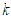different levels for the second factor, there are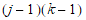dummy variables corresponding to the interactions. Hence, some interactions do not have a dummy variable associated with them in the linear regression, and a null reference may be returned even though valid interactions are specified.

This code prints out the intercept regression parameter, all factor regression parameters, and all interaction regression parameters:

Code Example – C# ANOVA

```Console.WriteLine( "Intercept" );
```
```Console.WriteLine( anova.RegressionInterceptParameter );
```
```Console.WriteLine();

```

```AnovaRegressionFactorParam[] factorParams =
```
```  anova.RegressionFactorParameters;
```
```for ( int i = 0; i < factorParams.Length; i++ )
```
```{
```
```  Console.WriteLine( factorParams[i].FactorLevel );
```
```  Console.WriteLine( factorParams[i] );
```
```  Console.WriteLine();
```
```}

```

```AnovaRegressionInteractionParam[] interactionParams =
```
```  anova.RegressionInteractionParameters;
```
```for ( int i = 0; i < interactionParams.Length; i++ )
```
```{
```
```  Console.WriteLine( interactionParams[i].FactorALevel +  " x " +
```
```                     interactionParams[i].FactorBLevel );
```
```  Console.WriteLine( interactionParams[i] );
```
```  Console.WriteLine();
```
```}
```

Code Example – VB ANOVA

```Console.WriteLine("Intercept")
```
```Console.WriteLine(Anova.RegressionInterceptParameter)
```
```Console.WriteLine()

```

```Dim FactorParams As AnovaRegressionFactorParam() =
```
```  Anova.RegressionFactorParameters
```
```For I As Integer = 0 To FactorParams.Length - 1
```
```  Console.WriteLine(FactorParams(I).FactorLevel)
```
```  Console.WriteLine(FactorParams(I))
```
```  Console.WriteLine()
```
```Next

```

```Dim InteractionParams As AnovaRegressionInteractionParam() =
```
```  Anova.RegressionInteractionParameters
```
```For I As Integer = 0 To InteractionParams.Length - 1
```
```  Console.WriteLine(InteractionParams(I).FactorALevel & " x " &
```
```InteractionParams(I).FactorBLevel)
```
```  Console.WriteLine(InteractionParams(I))
```
```  Console.WriteLine()
```
```Next
```

Example output:

```Intercept
```
```Value                        : 28.875
```
```Standard Error               : 1.63741694728596
```
```t-Statistic for parameter = 0: 17.6344821933477
```
```p-value for t-Statistic      : 8.35997937542743E-13
```
```0.05 confidence interval     : [2.5435E+001, 3.2315E+001]

```

```A1
```
```Value                        : 4.375
```
```Standard Error               : 1.63741694728596
```
```t-Statistic for parameter = 0: 2.67189124141632
```
```p-value for t-Statistic      : 0.0155516784650136
```
```0.05 confidence interval     : [9.3491E-001, 7.8151E+000]

```

```B1
```
```Value                        : 25.5
```
```Standard Error               : 2.31565725411135
```
```t-Statistic for parameter = 0: 11.0119923640365
```
```p-value for t-Statistic      : 1.98637151171965E-09
```
```0.05 confidence interval     : [2.0635E+001, 3.0365E+001]

```

```B2
```
```Value                        : -7.25
```
```Standard Error               : 2.31565725411135
```
```t-Statistic for parameter = 0: -3.13086057408882
```
```p-value for t-Statistic      : 0.00577563474636933
```
```0.05 confidence interval     : [-1.2115E+001, -2.3850E+000]

```

```A1 x B1
```
```Value                        : 6
```
```Standard Error               : 2.31565725411135
```
```t-Statistic for parameter = 0: 2.59105702683213
```
```p-value for t-Statistic      : 0.0184427158909004
```
```0.05 confidence interval     : [1.1350E+000, 1.0865E+001]

```

```A1 x B2
```
```Value                        : -0.999999999999999
```
```Standard Error               : 2.31565725411135
```
```t-Statistic for parameter = 0: -0.431842837805354
```
```p-value for t-Statistic      : 0.670984111233603
```
```0.05 confidence interval     : [-5.8650E+000, 3.8650E+000]
```

1. S. A. Glantz and B. K. Slinker, Primer of Applied Regression & Analysis of Variance (2nd ed.), NewYork, McGraw-Hill, 2001, pp. 357-358.

Top

Top